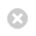Close

UCL Earth Sciences

Home# GEOL0005 Foundations of Physical Geoscience

This is a basic maths and physics course to prepare the student for their second year courses in our Earth Science programmes. It includes significant practical and problem work components.

Module details
TitleFoundations of Physical Geoscience
UG CodeGEOL0005
Other Contributors
Term1
Credit15 credits
Written Exam50%
Problem Sheets50%
Practical
Pre-RequisitesNone
Maths & Stats Content and RequirementGeneral GCSE-level (or equivalent) knowledge of maths
Total Number of Hours of Student Work188 hours
Hours of Lectures/Seminars20 hours
Hours of Practicals/Problem Classes30 hours
Hours of Tutorials0
Days of Fieldwork0
Categorizing Student PerformanceGEOL0005
Content

Topics studied in lectures and practicals include:

• Geometry and Co-ordinates (Measurement and geometry);
• Exponents and Graphs. (Graphical Methods);
• Dimensional Analysis and Mechanics 1. (Graphs and Computers);
• Mechanics 2 and Elasticity.(Determining 'g');
• Waves and Light. (The nature of light);
• Thermal and Transport Processes. (Viscosity);
• Electricity and Magnetism.(Electromagnetism);
• Differentiation and Integration.(Finite vs. Infinitesimal);
• Errors and Statistics.(Elementary Statistics).

#### AIMS

This course is intended to introduce Earth science students to the basic fundamental principles of mathematics and physics, assuming minimal previous knowledge. The student will be taught the essential tools of mathematics required for the geology, environmental geoscience and earth science degrees, and will study the basic principles of physics behind the fundamental processes occurring within the Earth and our solar system. Practical sessions and problem classes will illustrate the material covered within the lectures and will give the students training in good experimental techniques and scientific methods. In addition, the student will develop computer skills through exercises in word-processing, spreadsheet analysis and graph drawing.